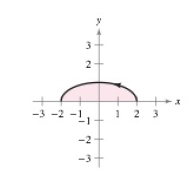Chapter 10, Problem 58RE

Chapter
Section
Textbook Problem

# Area In Exercises 57 and 58, find the area of the region. x = 2 cos θ y = sin θ 0 ≤ θ ≤ πTo determine

To calculate: The area of the region when curve is x=2cosθ, y=sinθ.

Explanation

Given:

The curves are x=2cosθ, y=sinθ and 0θπ.

Formula used:

The formula for area is A=abydx when ayb and x,y are functions.

The formula for differentiation is:

d(cosθ)dθ=sinθ

The identity:

sinθ=1cos2θ2

The formula for integration is:

cosaθdθ=sinaθa

dθ=θ

Calculation:

Consider the given equations:

x=2cosθ

And

y=sinθ

The formula for area is A=abydx when axb, ayb and x,y are functions

### Still sussing out bartleby?

Check out a sample textbook solution.

See a sample solution

#### The Solution to Your Study Problems

Bartleby provides explanations to thousands of textbook problems written by our experts, many with advanced degrees!

Get Started

#### Differentiate. y=x2+1

Single Variable Calculus: Early Transcendentals, Volume I

#### Find for the functions in Problems 7-10. 10.

Mathematical Applications for the Management, Life, and Social Sciences

#### Evaluate: 3211.

College Algebra (MindTap Course List)